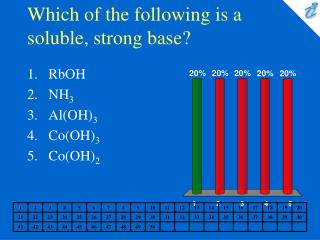DownloadDownload PresentationWhich of the following is a soluble, strong base?

# Which of the following is a soluble, strong base?

Télécharger la présentation## Which of the following is a soluble, strong base?

- - - - - - - - - - - - - - - - - - - - - - - - - - - E N D - - - - - - - - - - - - - - - - - - - - - - - - - - -
##### Presentation Transcript

1. Which of the following is a soluble, strong base? • RbOH • NH3 • Al(OH)3 • Co(OH)3 • Co(OH)2

2. Which salt is produced from a strong acid and a strong base? • NH4Cl • NaClO • KClO3 • Na2CO3 • LiNO2

3. Which solution should be the best conductor of electrical current because it would contain the largest number of ions in aqueous solution? • 0.10 M NH4NO3 • 0.10 M K2SO4 • 0.20 M BaSO4 • 0.10 M NaCl • 0.10 M HClO4

4. Consider a 0.020 M Fe2(SO4)3 solution. Which one of the responses includes all of the following statements that are correct, and no incorrect statements? I. The solution is 0.020 M in Fe3+. II. The solution is 0.030 M in SO42-. III. The total concentration of ions in the solution is 0.050 M. IV. The solution is 0.040 M in Fe3+. V. The solution is 0.060 M in SO42-. VI. The total concentration of ions in the solution is 0.10 M. • I and II • I, II, and III • IV and V • IV, V, and VI • none of these

5. The pH of a solution is 4.80. What is the concentration of hydroxide ions in this solution? • 4.2 x 10-9M • 1.6 x 10-5M • 3.6 x 10-12M • 6.3 x 10-10M • 2.0 x 10-8M

6. Calculate the pH of a solution that is 0.00030 M in Ba(OH)2. • 10.47 • 9.94 • 8.63 • 12.42 • 10.78

7. We add enough acid to a solution to cause the pH to decrease from 6.5 to 5.5. This means that • [OH-] increases by a factor of 10. • [H3O+] increases by a factor of 10. • [OH-] increases by 1 M. • [H3O+] increases by 1 M. • [H3O+] increases by a factor of 6.5/5.5.

8. Which response includes all of the following that are weak acids, and no strong acids? I. H2SO3 II. HClO III. H2SO4 IV. HClO3 V. HClO4 VI. C6H5COOH • I, II, and III • II, IV, V, and VI • I, II, and VI • IV, V, and VI • I and III

9. Calculate the pH of 0.020 M HOBr solution. Ka= 2.5 x 10-9 • 4.80 • 4.91 • 5.02 • 5.15 • 5.38

10. Calculate the original molarity of a solution of acetic acid that is 3.0% ionized. Ka = 1.8 x 10-5 • 0.076 M • 1.9 x 10-2M • 0.038 M • 3.4 x 10-4M • 6.7 x 10-4M

11. Trimethylamine ionizes as follows in water. What is the percent ionization for a 9.0 x 10-2 M solution of (CH3)3N? Kb = 7.4 x 10-5 (CH3)3N + H2O {image} (CH3)3NH+ + OH- • 2.9% • 0.030% • 0.18% • 0.42% • 5.8%

12. Which of the following anions is the strongest base? • Cl- • NO3- • ClO4- • F- • Br-

13. Which response includes all of the following salts that give neutral aqueous solutions, and no other salts? I. KNO3 II. BaCl2 III. NaCH3COO IV. NH4Cl V. NaBr • I and II • I, II, III, and V • III and IV • IV and V • I, II, and V

14. What is the value of the (base) hydrolysis constant for NaBO2, sodium metaborate? • 3.2 x 10-6 • 1.7 x 10-5 • 1.8 x 10-6 • 6.0 x 104 • 2.2 x 10-7

15. What is the pH of 0.25 M KCN solution? • 1.17 • 2.60 • 10.42 • 11.40 • 12.83

16. What is the percent hydrolysis in 0.20 M NaNO2 solution? • 0.0064% • 0.0047% • 0.0010% • 0.00061% • 0.00092%

17. Calculate the [H3O+] in a 0.20 M KCN solution. • 2.2 x 10-3M • 1.0 x 10-7M • 2.4 x 10-11M • 4.5 x 10-12M • 3.0 x 10-12M

18. The hydrolysis constant that would be used to calculate the pH in NH4Cl solution is given by • Kw/Ka (HCl) • Kw/Ka(Cl-) • Kw/Kb(NH3) • Kw/Kb(NH4+) • Kw/Kb(NH4Cl)

19. Calculate the pH of 0.050 M NH4Cl. • 4.62 • 4.84 • 5.04 • 5.16 • 5.28

20. Calculate the pH of 0.15 M Co(NO3)2. • 4.52 • 4.74 • 4.88 • 5.06 • 5.28